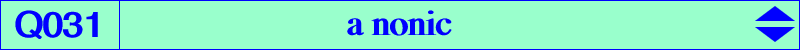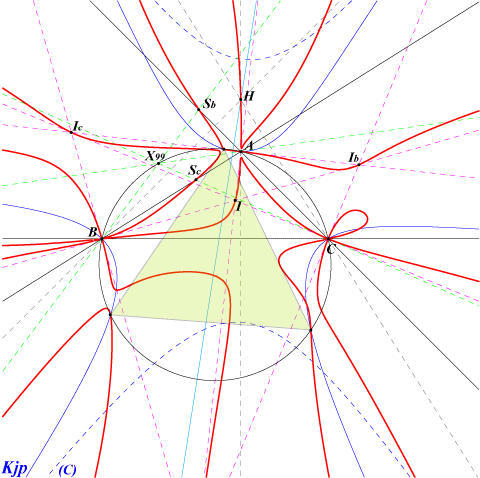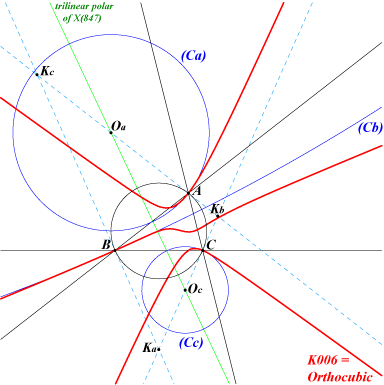too complicated to be written here. Click on the link to download a text file.X(1), X(4) excenters vertices of the circumtangential triangle Sa, Sb, Sc cevians of X(99)Q031 is the locus of pivots of all isogonal pK such that the centers Oa, Ob, Oc of the three osculating circles (Ca), (Cb), (Cc) at A, B, C are collinear. Q031 contains A, B, C which are triple points. The tangents at A are the two bisectors and the line passing through the reflection in the midpoint of BC of the trace Sa on BC of the trilinear polar of X(99), the Steiner point. Q031 meets BC again at Sa and two other always imaginary points lying on the conic (C) centered at K with equation : b^2c^2x^2+2a^2SAyz + cyclic = 0. Similarly for B and C. Q031 also contains the four in/excenters for which the pK degenerates into three bisectors and H for which the pK is the Orthocubic.